# Python Program to Find the Size of a File

This article was created to cover some programs in Python that find and print the size of a file entered by the user at run-time. Here is a list of programs covered in this article:

• Find the size of a file using the getsize() function.
• Find the size of a file without using any pre-defined function.
• Find the size of a file in KB, MB, GB, or TB.

## Find the size of a file using getsize()

The question is: write a Python program that finds the size of a file using the getsize() method. The program given below is the answer to this question:

```import os

print("Enter the Name of File: ")
fileName = input()
sizeOfFile = os.path.getsize(fileName)
print("\nSize of Given File (in bytes) is:")
print(sizeOfFile)```

Here is its sample run:Now supply the input as the name of the file to find and print its size. Here is its sample output with user input codescracker.txt. This file already exists in the current directory. That is, the folder where the above program (source code) is also saved: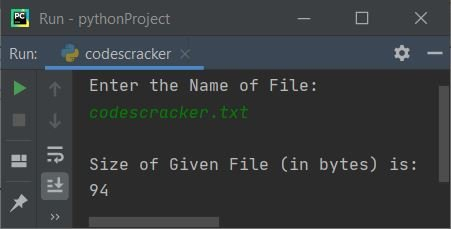Here is the window that shows the details along with the size of the same file as provided in the sample run: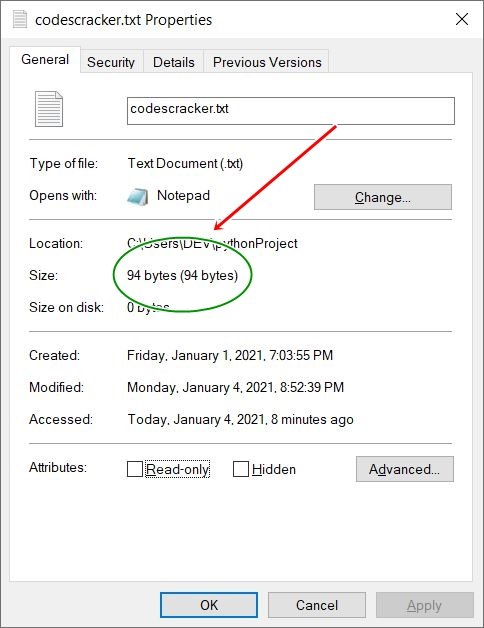And the snapshot given below shows the content of the same file: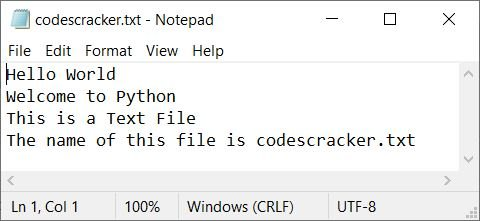Note: As you can see from the content of the file codescracker.txt, There are 92 characters (with spaces) and 2 newlines (that cannot be seen). The second and third lines of text have newlines. That is, after the first line, there is a newline, and then after the second line, there is a newline.

## Find File Size Using User-Based Code

The question is: write a program in Python that finds and prints the size of a file without using any pre-defined function. The following program is the answer to this question:

```print(end="Enter File's Name: ")
fileName = input()
try:
fileHandle = open(fileName, "r")
sizeOfFile = 0
sizeOfFile = sizeOfFile + (len(line)+1)
sizeOfFile = sizeOfFile-1
print("\nFile's Size = " +str(sizeOfFile)+ " bytes")
except IOError:
print("\nThe File doesn't exist!")```

Here is its sample run with the same file name, say codescracker.txt: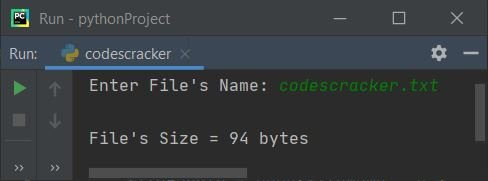Here is another sample run with user input, codescracker.py (the name of the current Python program's source code):## Find the file size in KB, MB, GB, or TB

Now this is the final program of this article that finds and prints the size of the file in KB, MB, GB, and TB. Let's have a look at the program and its sample output:

```print(end="Enter File's Name: ")
fileName = input()
try:
fileHandle = open(fileName, "r")
sizeOfFile = 0
sizeOfFile = sizeOfFile + (len(line)+1)
sizeOfFile = sizeOfFile-1
kb = sizeOfFile/1024
if kb>1:
mb = kb/1024
if mb>1:
gb = mb/1024
if gb>1:
tb = gb/1024
if tb>1:
tb = "{0:.2f}".format(tb)
print("\nFile's Size = " +str(tb)+ " TB")
else:
gb = "{0:.2f}".format(gb)
print("\nFile's Size = " +str(gb)+ " GB")
else:
mb = "{0:.2f}".format(mb)
print("\nFile's Size = " +str(mb)+ " MB")
else:
kb = "{0:.2f}".format(kb)
print("\nFile's Size = " +str(kb)+ " KB")
else:
print("\nFile's Size = " +str(sizeOfFile)+ " bytes")
except IOError:
print("\nThe File doesn't exist!")```

Here is its sample run with user input, codes.txt: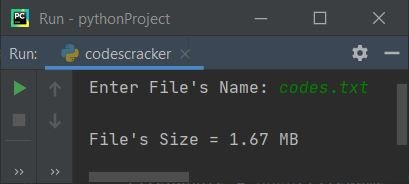Here is a snapshot of the properties of the codes.txt file: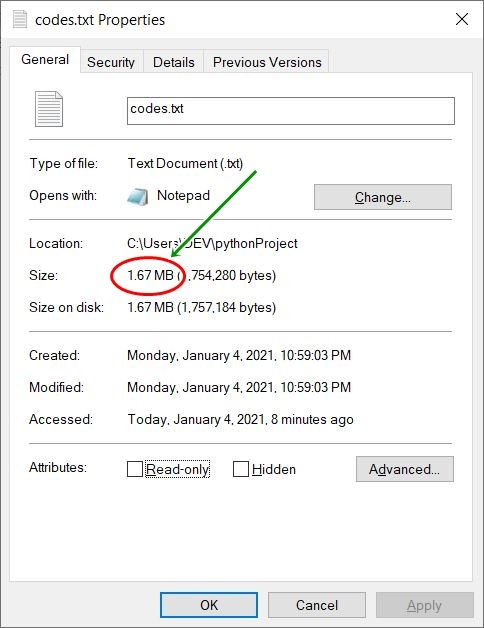Python Online Test

« Previous Program Next Program »